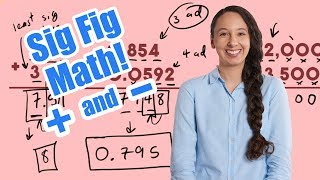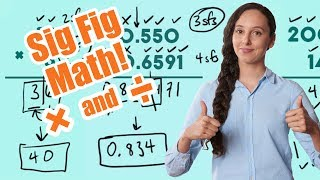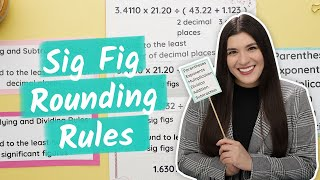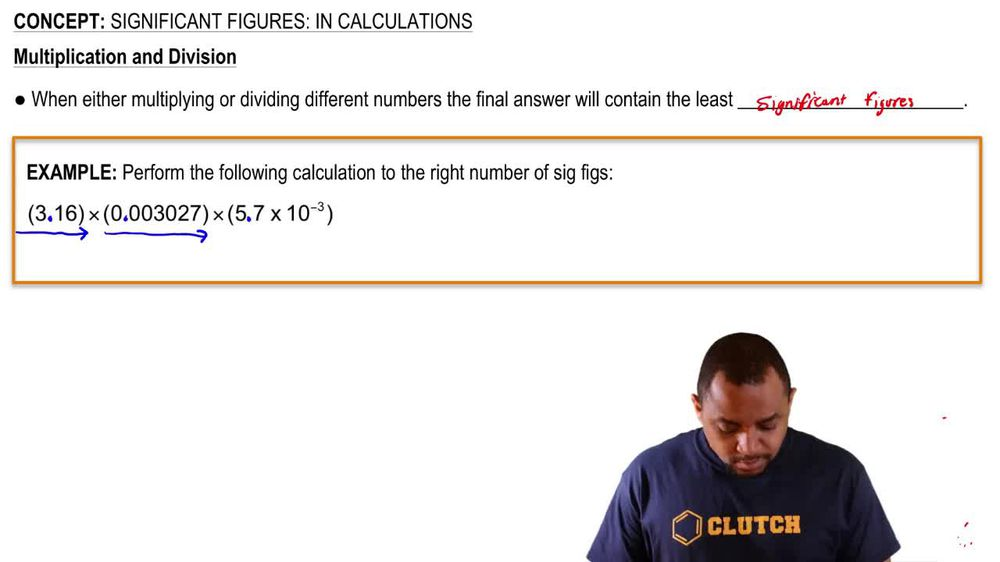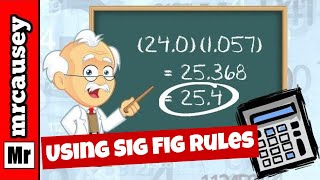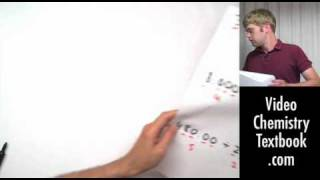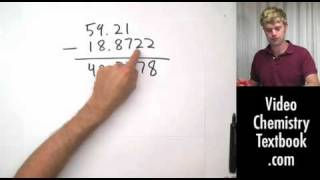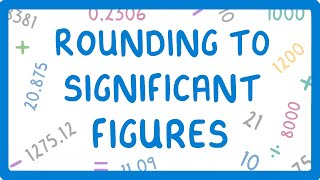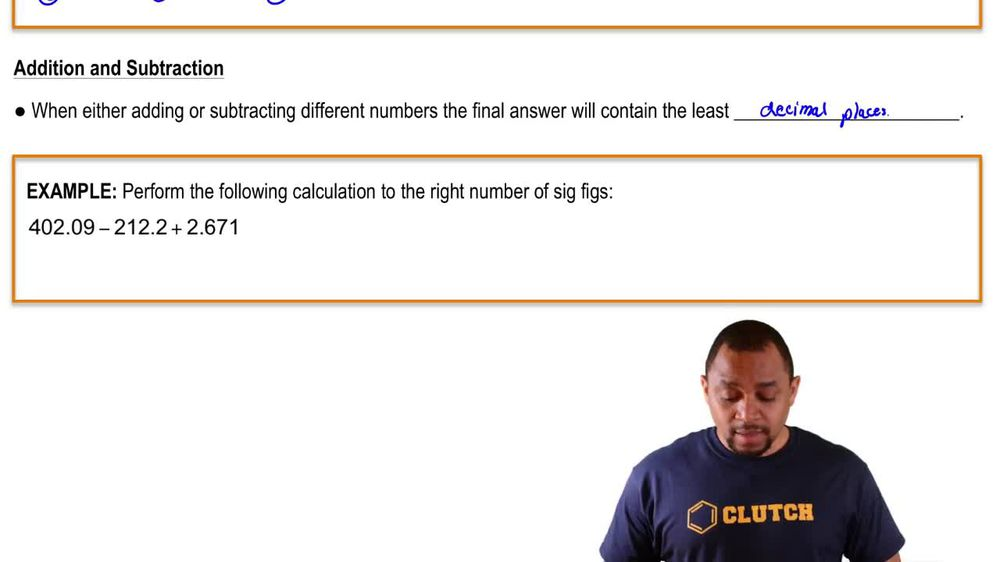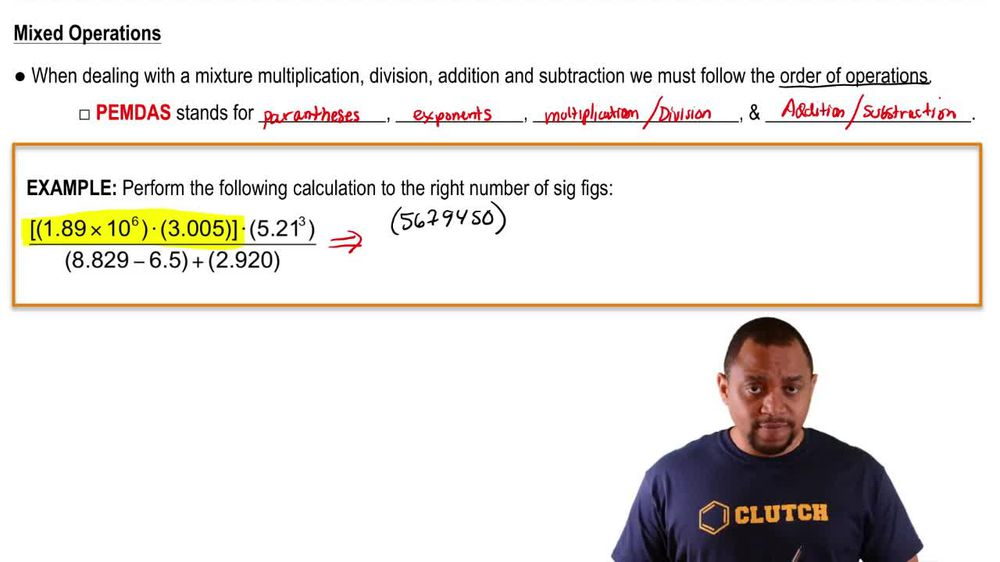Start typing, then use the up and down arrows to select an option from the list.
1. 1. Intro to General Chemistry2. Significant Figures: In Calculations
Problem

# Calculate to the correct number of significant figures. a. 89.3 * 77.0 * 0.08 b. (5.01 * 105) , (7.8 * 102) c. 4.005 * 74 * 0.007 d. 453 , 2.031

Relevant Solution1m
Play a video:
Hi everyone here, we have a question asking us to solve the problem using the correct number of significant figures. 6.1830 times in parentheses, 14.598 divided by 3.1. So let's rewrite our problem here and we're going to solve what's in parentheses first, of course, So that equals 4.7 And now that equals .0601. So now we have to get it to the correct number of significant figures When multiplying and dividing the correct number of significant figures is the number with the least significant figures, which in this case is our 3.1 With two significant figures. So we only want two significant figures, which means we're going to look at our nine here and we're going to look at the right of it. And if it's above five we'll round up and if it's below five we will round down and it is a zero. So we will be rounding down to and that is our final answer. Thank you for watching. Bye.